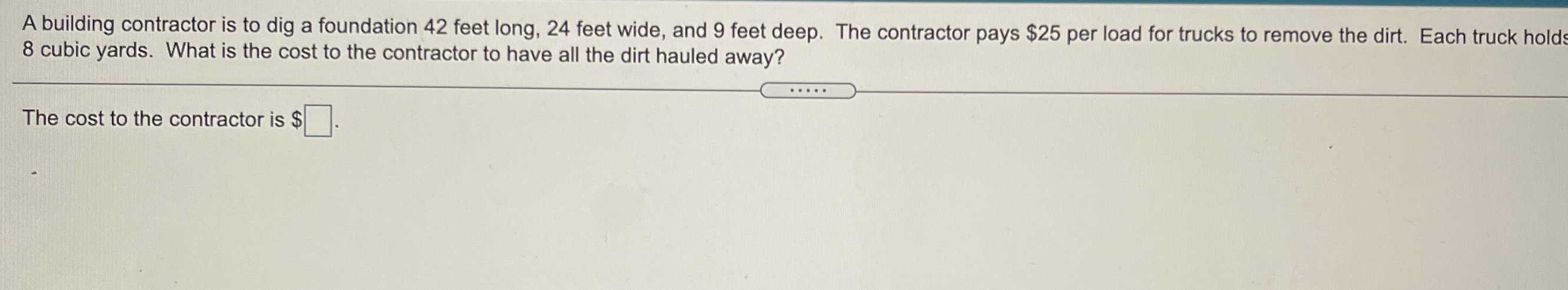### ¿Todavía tienes preguntas de matemáticas?

Pregunte a nuestros tutores expertos
Algebra
PreguntaA building contractor is to dig a foundation $$42$$ feet long, $$24$$ feet wide, and $$9$$ feet deep. The contractor pays $$\ 25$$ per load for trucks to remove the dirt. Each truck holds $$8$$ cubic yards. What is the cost to the contractor to have all the dirt hauled away?

The cost to the contractor is $$\ \square$$ .